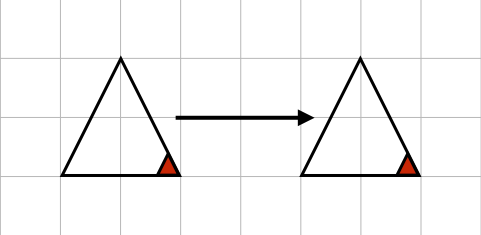# 4.4: Transformations

$$\newcommand{\vecs}{\overset { \rightharpoonup} {\mathbf{#1}} }$$ $$\newcommand{\vecd}{\overset{-\!-\!\rightharpoonup}{\vphantom{a}\smash {#1}}}$$$$\newcommand{\id}{\mathrm{id}}$$ $$\newcommand{\Span}{\mathrm{span}}$$ $$\newcommand{\kernel}{\mathrm{null}\,}$$ $$\newcommand{\range}{\mathrm{range}\,}$$ $$\newcommand{\RealPart}{\mathrm{Re}}$$ $$\newcommand{\ImaginaryPart}{\mathrm{Im}}$$ $$\newcommand{\Argument}{\mathrm{Arg}}$$ $$\newcommand{\norm}{\| #1 \|}$$ $$\newcommand{\inner}{\langle #1, #2 \rangle}$$ $$\newcommand{\Span}{\mathrm{span}}$$ $$\newcommand{\id}{\mathrm{id}}$$ $$\newcommand{\Span}{\mathrm{span}}$$ $$\newcommand{\kernel}{\mathrm{null}\,}$$ $$\newcommand{\range}{\mathrm{range}\,}$$ $$\newcommand{\RealPart}{\mathrm{Re}}$$ $$\newcommand{\ImaginaryPart}{\mathrm{Im}}$$ $$\newcommand{\Argument}{\mathrm{Arg}}$$ $$\newcommand{\norm}{\| #1 \|}$$ $$\newcommand{\inner}{\langle #1, #2 \rangle}$$ $$\newcommand{\Span}{\mathrm{span}}$$$$\newcommand{\AA}{\unicode[.8,0]{x212B}}$$

## Rigid Motions

### Translations

A translation is a transformation that moves a figure (without altering dimensions) to a new position.

Example $$\PageIndex{1}$$:### Reflections

A reflection is a transformation that maps a figure so that a line, called the line of reflection, is the perpendicular bisector of every line segment joining a point on the figure and the corresponding point on the reflected figure

Example $$\PageIndex{2}$$:### Rotations

Rotations are transformations where a figure is rotated about its center by a specified amount (given usually in degrees).

Example $$\PageIndex{3}$$:Thinking Out Loud:

Are reflections, translations, and rotations related?

How are the operations of translation, reflection, and rotation like the arithmetic operations?

## Composite transformations

### Glide reflections

Glide reflections are a translation followed by a reflection with the condition that the translation vector and the line of reflection are parallel (that is, point in the same direction).

Example $$\PageIndex{4}$$:Example $$\PageIndex{5}$$:

Consider the equilateral triangle below. What rigid motions can we do to it that will result in the triangle occupying the same space? What happens if we combine rigid motions? Keep in mind that "doing nothing" is also a rigid motion.Exercise $$\PageIndex{1}$$

Consider a square. What rigid motions can we do to it that will result in the square occupying the same space? What happens if we combine rigid motions? Keep in mind that "doing nothing" is also a rigid motion.